xPartition function (statistical mechanics)Encyclopedia
Partition functions describe the statistical
Statistics
Statistics is the study of the collection, organization, analysis, and interpretation of data. It deals with all aspects of this, including the planning of data collection in terms of the design of surveys and experiments....

properties of a system in thermodynamic equilibrium
Thermodynamic equilibrium
In thermodynamics, a thermodynamic system is said to be in thermodynamic equilibrium when it is in thermal equilibrium, mechanical equilibrium, radiative equilibrium, and chemical equilibrium. The word equilibrium means a state of balance...

. It is a function
Function (mathematics)
In mathematics, a function associates one quantity, the argument of the function, also known as the input, with another quantity, the value of the function, also known as the output. A function assigns exactly one output to each input. The argument and the value may be real numbers, but they can...

of temperature
Temperature
Temperature is a physical property of matter that quantitatively expresses the common notions of hot and cold. Objects of low temperature are cold, while various degrees of higher temperatures are referred to as warm or hot...

and other parameters, such as the volume
Volume
Volume is the quantity of three-dimensional space enclosed by some closed boundary, for example, the space that a substance or shape occupies or contains....

enclosing a gas. Most of the aggregate thermodynamic
Thermodynamics
Thermodynamics is a physical science that studies the effects on material bodies, and on radiation in regions of space, of transfer of heat and of work done on or by the bodies or radiation...

variables of the system, such as the total energy
Energy
In physics, energy is an indirectly observed quantity. It is often understood as the ability a physical system has to do work on other physical systems...

, free energy
Thermodynamic free energy
The thermodynamic free energy is the amount of work that a thermodynamic system can perform. The concept is useful in the thermodynamics of chemical or thermal processes in engineering and science. The free energy is the internal energy of a system less the amount of energy that cannot be used to...

, entropy
Entropy
Entropy is a thermodynamic property that can be used to determine the energy available for useful work in a thermodynamic process, such as in energy conversion devices, engines, or machines. Such devices can only be driven by convertible energy, and have a theoretical maximum efficiency when...

, and pressure
Pressure
Pressure is the force per unit area applied in a direction perpendicular to the surface of an object. Gauge pressure is the pressure relative to the local atmospheric or ambient pressure.- Definition :...

, can be expressed in terms of the partition function or its derivative
Derivative
In calculus, a branch of mathematics, the derivative is a measure of how a function changes as its input changes. Loosely speaking, a derivative can be thought of as how much one quantity is changing in response to changes in some other quantity; for example, the derivative of the position of a...

s.

There are actually several different types of partition functions, each corresponding to different types of statistical ensemble (or, equivalently, different types of free energy
Thermodynamic free energy
The thermodynamic free energy is the amount of work that a thermodynamic system can perform. The concept is useful in the thermodynamics of chemical or thermal processes in engineering and science. The free energy is the internal energy of a system less the amount of energy that cannot be used to...

.) The canonical partition function applies to a canonical ensemble
Canonical ensemble
The canonical ensemble in statistical mechanics is a statistical ensemble representing a probability distribution of microscopic states of the system...

, in which the system is allowed to exchange heat
Heat
In physics and thermodynamics, heat is energy transferred from one body, region, or thermodynamic system to another due to thermal contact or thermal radiation when the systems are at different temperatures. It is often described as one of the fundamental processes of energy transfer between...

with the environment at fixed temperature, volume, and number of particles. The grand canonical partition function applies to a grand canonical ensemble
Grand canonical ensemble
In statistical mechanics, a grand canonical ensemble is a theoretical collection of model systems put together to mirror the calculated probability distribution of microscopic states of a given physical system which is being maintained in a given macroscopic state...

, in which the system can exchange both heat and particles with the environment, at fixed temperature, volume, and chemical potential
Chemical potential
Chemical potential, symbolized by μ, is a measure first described by the American engineer, chemist and mathematical physicist Josiah Willard Gibbs. It is the potential that a substance has to produce in order to alter a system...

. Other types of partition functions can be defined for different circumstances; see partition function (mathematics)
Partition function (mathematics)
The partition function or configuration integral, as used in probability theory, information science and dynamical systems, is an abstraction of the definition of a partition function in statistical mechanics. It is a special case of a normalizing constant in probability theory, for the Boltzmann...

for generalizations.

### Definition

As a beginning assumption, assume that a thermodynamically large system is in constant thermal contact with the environment, with a temperature  T , and both the volume of the system and the number of constituent particles fixed. This kind of system is called a canonical ensemble
Canonical ensemble
The canonical ensemble in statistical mechanics is a statistical ensemble representing a probability distribution of microscopic states of the system...

. Let us label with  s  ( s = 1, 2, 3, ...) the exact states (microstates
Microstate (statistical mechanics)
In statistical mechanics, a microstate is a specific microscopic configuration of a thermodynamic system that the system may occupy with a certain probability in the course of its thermal fluctuations...

) that the system can occupy, and denote the total energy of the system when it is in microstate  s  as  Es . Generally, these microstates can be regarded as analogous to discrete quantum states of the system.

The canonical partition function is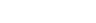,

where the "inverse temperature", β, is conventionally defined as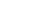with  kB  denoting Boltzmann's constant. The term  exp(–β·Es)  is known as the Boltzmann factor
Boltzmann factor
In physics, the Boltzmann factor is a weighting factor that determines the relative probability of a particle to be in a state i in a multi-state system in thermodynamic equilibrium at temperature T...

. In systems with multiple quantum states  s  sharing the same  Es , it is said that the energy levels of the system are degenerate. In the case of degenerate energy levels, we can write the partition function in terms of the contribution from energy levels (indexed by  j ) as follows: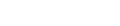,

where  gj  is the degeneracy factor, or number of quantum states  s  which have the same energy level defined by  Ej = Es .

The above treatment applies to quantum statistical mechanics
Statistical mechanics
Statistical mechanics or statistical thermodynamicsThe terms statistical mechanics and statistical thermodynamics are used interchangeably...

, where a physical system inside a finite-sized box will typically have a discrete set of energy
Energy
In physics, energy is an indirectly observed quantity. It is often understood as the ability a physical system has to do work on other physical systems...

eigenstates, which we can use as the states  s  above. In classical statistical mechanics, it is not really correct to express the partition function as a sum of discrete terms, as we have done. In classical mechanics
Classical mechanics
In physics, classical mechanics is one of the two major sub-fields of mechanics, which is concerned with the set of physical laws describing the motion of bodies under the action of a system of forces...

, the position and momentum variables of a particle can vary continuously, so the set of microstates is actually uncountable
Uncountable set
In mathematics, an uncountable set is an infinite set that contains too many elements to be countable. The uncountability of a set is closely related to its cardinal number: a set is uncountable if its cardinal number is larger than that of the set of all natural numbers.-Characterizations:There...

. In this case we must describe the partition function using an integral
Integral
Integration is an important concept in mathematics and, together with its inverse, differentiation, is one of the two main operations in calculus...

rather than a sum. For instance, the partition function of a gas of  N  identical classical particles is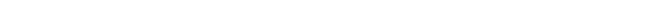where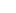indicate particle momenta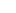indicate particle positions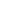is a shorthand notation serving as a reminder that theandare vectors in three dimensional space, and
H  is the classical Hamiltonian
Hamiltonian mechanics
Hamiltonian mechanics is a reformulation of classical mechanics that was introduced in 1833 by Irish mathematician William Rowan Hamilton.It arose from Lagrangian mechanics, a previous reformulation of classical mechanics introduced by Joseph Louis Lagrange in 1788, but can be formulated without...

.

The reason for the  N!  factor is discussed below. For simplicity, we will use the discrete form of the partition function in this article. Our results will apply equally well to the continuous form. The extra constant factor introduced in the denominator was introduced because, unlike the discrete form, the continuous form shown above is not dimensionless. To make it into a dimensionless quantity, we must divide it by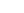where  h  is some quantity with units of action
Action (physics)
In physics, action is an attribute of the dynamics of a physical system. It is a mathematical functional which takes the trajectory, also called path or history, of the system as its argument and has a real number as its result. Action has the dimension of energy × time, and its unit is...

(usually taken to be Planck's constant).

In quantum mechanics, the partition function can be more formally written as a trace
Trace (linear algebra)
In linear algebra, the trace of an n-by-n square matrix A is defined to be the sum of the elements on the main diagonal of A, i.e.,...

over the state space
Mathematical formulation of quantum mechanics
The mathematical formulations of quantum mechanics are those mathematical formalisms that permit a rigorous description of quantum mechanics. Such are distinguished from mathematical formalisms for theories developed prior to the early 1900s by the use of abstract mathematical structures, such as...

(which is independent of the choice of basis
Basis (linear algebra)
In linear algebra, a basis is a set of linearly independent vectors that, in a linear combination, can represent every vector in a given vector space or free module, or, more simply put, which define a "coordinate system"...

):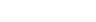,

where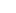is the quantum Hamiltonian operator
Hamiltonian (quantum mechanics)
In quantum mechanics, the Hamiltonian H, also Ȟ or Ĥ, is the operator corresponding to the total energy of the system. Its spectrum is the set of possible outcomes when one measures the total energy of a system...

. The exponential of an operator can be defined using the exponential power series
Characterizations of the exponential function
In mathematics, the exponential function can be characterized in many ways. The following characterizations are most common. This article discusses why each characterization makes sense, and why the characterizations are independent of and equivalent to each other...

.
The classical form of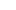is recovered when the trace is expressed in terms
of coherent state
Coherent state
In quantum mechanics a coherent state is a specific kind of quantum state of the quantum harmonic oscillator whose dynamics most closely resembles the oscillating behaviour of a classical harmonic oscillator...

s

and when quantum-mechanical uncertainties
Uncertainty principle
In quantum mechanics, the Heisenberg uncertainty principle states a fundamental limit on the accuracy with which certain pairs of physical properties of a particle, such as position and momentum, can be simultaneously known...

in the position and momentum of a particle
are regarded as negligible. Formally, one inserts under the trace for each degree of freedom
a resolution of the identity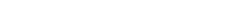where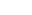is a normalised Gaussian wavepacket centered at
positionand momentum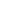. Thus,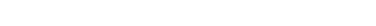A coherent state is an approximate eigenstate
of both operators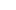and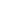,
hence also of the Hamiltonian, with errors of the size of
the uncertainties. If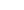and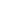can be regarded as
zero, the action ofreduces to multiplication by the classical
Hamiltonian, andreduces to the classical configuration integral.

### Meaning and significance

It may not be obvious why the partition function, as we have defined it above, is an important quantity. First, let us consider what goes into it. The partition function is a function of the temperature T and the microstate energies E1, E2, E3, etc. The microstate energies are determined by other thermodynamic variables, such as the number of particles and the volume, as well as microscopic quantities like the mass of the constituent particles. This dependence on microscopic variables is the central point of statistical mechanics. With a model of the microscopic constituents of a system, one can calculate the microstate energies, and thus the partition function, which will then allow us to calculate all the other thermodynamic properties of the system.

The partition function can be related to thermodynamic properties because it has a very important statistical meaning. The probability Ps that the system occupies microstate s is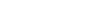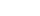is the well-known Boltzmann factor
Boltzmann factor
In physics, the Boltzmann factor is a weighting factor that determines the relative probability of a particle to be in a state i in a multi-state system in thermodynamic equilibrium at temperature T...

. (For a detailed derivation of this result, see canonical ensemble
Canonical ensemble
The canonical ensemble in statistical mechanics is a statistical ensemble representing a probability distribution of microscopic states of the system...

.) The partition function thus plays the role of a normalizing constant (note that it does not depend on s), ensuring that the probabilities sum up to one: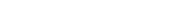This is the reason for calling Z the "partition function": it encodes how the probabilities are partitioned among the different microstates, based on their individual energies. The letter Z stands for the German
German language
German is a West Germanic language, related to and classified alongside English and Dutch. With an estimated 90 – 98 million native speakers, German is one of the world's major languages and is the most widely-spoken first language in the European Union....

word Zustandssumme, "sum over states". This notation also implies another important meaning of the partition function of a system: it counts the (weighted) number of states a system can occupy. Hence if all states are equally probable (equal energies) the partition function is the total number of possible states. Often this is the practical importance of Z.

### Calculating the thermodynamic total energy

In order to demonstrate the usefulness of the partition function, let us calculate the thermodynamic value of the total energy. This is simply the expected value
Expected value
In probability theory, the expected value of a random variable is the weighted average of all possible values that this random variable can take on...

, or ensemble average
Ensemble average
In statistical mechanics, the ensemble average is defined as the mean of a quantity that is a function of the micro-state of a system , according to the distribution of the system on its micro-states in this ensemble....

for the energy, which is the sum of the microstate energies weighted by their probabilities: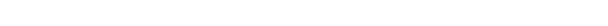or, equivalently,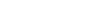Incidentally, one should note that if the microstate energies depend on a parameter λ in the manner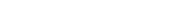then the expected value of A is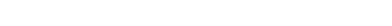This provides us with a method for calculating the expected values of many microscopic quantities. We add the quantity artificially to the microstate energies (or, in the language of quantum mechanics, to the Hamiltonian), calculate the new partition function and expected value, and then set λ to zero in the final expression. This is analogous to the source field method used in the path integral formulation
Path integral formulation
The path integral formulation of quantum mechanics is a description of quantum theory which generalizes the action principle of classical mechanics...

of quantum field theory
Quantum field theory
Quantum field theory provides a theoretical framework for constructing quantum mechanical models of systems classically parametrized by an infinite number of dynamical degrees of freedom, that is, fields and many-body systems. It is the natural and quantitative language of particle physics and...

.

### Relation to thermodynamic variables

In this section, we will state the relationships between the partition function and the various thermodynamic parameters of the system. These results can be derived using the method of the previous section and the various thermodynamic relations.

As we have already seen, the thermodynamic energy is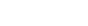The variance
Variance
In probability theory and statistics, the variance is a measure of how far a set of numbers is spread out. It is one of several descriptors of a probability distribution, describing how far the numbers lie from the mean . In particular, the variance is one of the moments of a distribution...

in the energy (or "energy fluctuation") is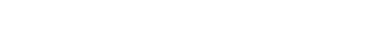The heat capacity
Heat capacity
Heat capacity , or thermal capacity, is the measurable physical quantity that characterizes the amount of heat required to change a substance's temperature by a given amount...

is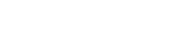The entropy
Entropy
Entropy is a thermodynamic property that can be used to determine the energy available for useful work in a thermodynamic process, such as in energy conversion devices, engines, or machines. Such devices can only be driven by convertible energy, and have a theoretical maximum efficiency when...

is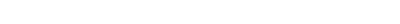where A is the Helmholtz free energy
Helmholtz free energy
In thermodynamics, the Helmholtz free energy is a thermodynamic potential that measures the “useful” work obtainable from a closed thermodynamic system at a constant temperature and volume...

defined as A = U - TS, where U= is the total energy and S is the entropy
Entropy
Entropy is a thermodynamic property that can be used to determine the energy available for useful work in a thermodynamic process, such as in energy conversion devices, engines, or machines. Such devices can only be driven by convertible energy, and have a theoretical maximum efficiency when...

, so that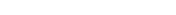### Partition functions of subsystems

Suppose a system is subdivided into N sub-systems with negligible interaction energy. If the partition functions of the sub-systems are ζ1, ζ2, ..., ζN, then the partition function of the entire system is the product of the individual partition functions: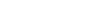If the sub-systems have the same physical properties, then their partition functions are equal, ζ1 = ζ2 = ... = ζ, in which case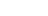However, there is a well-known exception to this rule. If the sub-systems are actually identical particles
Identical particles
Identical particles, or indistinguishable particles, are particles that cannot be distinguished from one another, even in principle. Species of identical particles include elementary particles such as electrons, and, with some clauses, composite particles such as atoms and molecules.There are two...

, in the quantum mechanical
Quantum mechanics
Quantum mechanics, also known as quantum physics or quantum theory, is a branch of physics providing a mathematical description of much of the dual particle-like and wave-like behavior and interactions of energy and matter. It departs from classical mechanics primarily at the atomic and subatomic...

sense that they are impossible to distinguish even in principle, the total partition function must be divided by a N ! (N factorial
Factorial
In mathematics, the factorial of a non-negative integer n, denoted by n!, is the product of all positive integers less than or equal to n...

):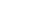This is to ensure that we do not "over-count" the number of microstates. While this may seem like a strange requirement, it is actually necessary to preserve the existence of a thermodynamic limit for such systems. This is known as the Gibbs paradox
In statistical mechanics, a semi-classical derivation of the entropy that doesn't take into account the indistinguishability of particles, yields an expression for the entropy which is not extensive...

.

### Definition

In a manner similar to the definition of the canonical partition function for the canonical ensemble
Canonical ensemble
The canonical ensemble in statistical mechanics is a statistical ensemble representing a probability distribution of microscopic states of the system...

, we can define a grand canonical partition function for a grand canonical ensemble
Grand canonical ensemble
In statistical mechanics, a grand canonical ensemble is a theoretical collection of model systems put together to mirror the calculated probability distribution of microscopic states of a given physical system which is being maintained in a given macroscopic state...

, a system that can exchange both heat and particles with the environment, which has a constant temperature  T , and a chemical potential
Chemical potential
Chemical potential, symbolized by μ, is a measure first described by the American engineer, chemist and mathematical physicist Josiah Willard Gibbs. It is the potential that a substance has to produce in order to alter a system...

μ . The grand canonical partition function, although conceptually more involved, simplifies the theoretical handling of quantum systems because it incorporates in a simple way the spin-statistics of the particles (i.e. whether particles are bosons or fermions). A canonical partition function, which has a given number of particles is in fact difficult to write down because of spin statistics.

The grand canonical partition function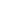for an ideal quantum gas (a gas of non-interacting particles in a given potential well) is given by the following expression: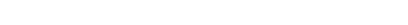where  N  is the total number of particles in the gas, index  i  runs over every microstate (that is, a single particle state in the potential) with  ni  being the number of particles occupying microstate  i  and εi being the energy of a particle in that microstate. The set  { ni }  is the collection of all possible occupation numbers for each of these microstates such that  Σ ni = N .

For example, consider the  N = 3  term in the above sum. One possible set of occupation numbers would be  ni = 0, 1, 0, 2, 0 ...  and the contribution of this set of occupation numbers to the  N = 3  term would be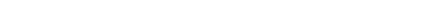.

For bosons, the occupation numbers can take any integer values as long as their sum is equal to  N . For fermions, the Pauli exclusion principle
Pauli exclusion principle
The Pauli exclusion principle is the quantum mechanical principle that no two identical fermions may occupy the same quantum state simultaneously. A more rigorous statement is that the total wave function for two identical fermions is anti-symmetric with respect to exchange of the particles...

requires that the occupation numbers only be  0  or  1 , again adding up to  N .

### Specific expressions

The above expression for the grand partition function can be shown to be
mathematically equivalent to: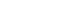.

(The above product is sometimes taken over all states with equal energy, rather than over each state, in which case the individual partition functions must be raised to a power  gi  where  gi  is the number of such states.  gi  is also referred to as the "degeneracy" of states.)

For a system composed of boson
Boson
In particle physics, bosons are subatomic particles that obey Bose–Einstein statistics. Several bosons can occupy the same quantum state. The word boson derives from the name of Satyendra Nath Bose....

s: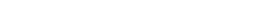and for a system composed of fermion
Fermion
In particle physics, a fermion is any particle which obeys the Fermi–Dirac statistics . Fermions contrast with bosons which obey Bose–Einstein statistics....

s: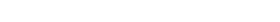.

For the case of a Maxwell-Boltzmann gas, we must use "correct Boltzmann counting" and divide the Boltzmann factor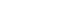by  ni! .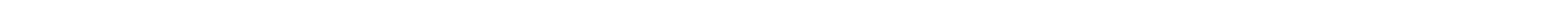,

where  α = –β·μ .

For Boltzmann particles this yields: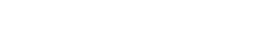.

which are just the results found using the canonical ensemble for
Maxwell-Boltzmann statistics, Bose-Einstein statistics and Fermi-Dirac statistics
Fermi-Dirac statistics
Fermi–Dirac statistics is a part of the science of physics that describes the energies of single particles in a system comprising many identical particles that obey the Pauli Exclusion Principle...

, respectively. (The degeneracy  gi  is missing from the above equations because the index  i  is summing over individual microstates rather than energy eigenvalues.)
\frac{1}{\beta}\left(\frac{\partial\ln(\mathcal{Z})}{\partial \mu} \right)_{\beta,V}.
.

#### VarianceVarianceIn probability theory and statistics, the variance is a measure of how far a set of numbers is spread out. It is one of several descriptors of a probability distribution, describing how far the numbers lie from the mean . In particular, the variance is one of the moments of a distribution... in total number of particles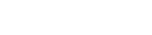.

#### Internal energy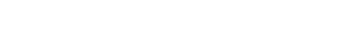.

#### VarianceVarianceIn probability theory and statistics, the variance is a measure of how far a set of numbers is spread out. It is one of several descriptors of a probability distribution, describing how far the numbers lie from the mean . In particular, the variance is one of the moments of a distribution... in internal energyInternal energyIn thermodynamics, the internal energy is the total energy contained by a thermodynamic system. It is the energy needed to create the system, but excludes the energy to displace the system's surroundings, any energy associated with a move as a whole, or due to external force fields. Internal...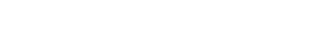.

#### Mechanical equation of stateEquation of stateIn physics and thermodynamics, an equation of state is a relation between state variables. More specifically, an equation of state is a thermodynamic equation describing the state of matter under a given set of physical conditions...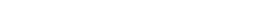.

### Relation to potential V

For the case of a non-interacting gas, using the "Semiclassical Approach" we can write (approximately) the inverse of the potential in the form: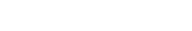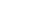(valid for high T )

supposing that the Hamiltonian of every particle is H=T+V .

### Discussion

Before specific results can be obtained from the grand canonical partition function, the energy levels of the system under consideration need to be specified. For example, the particle in a box
Particle in a box
In quantum mechanics, the particle in a box model describes a particle free to move in a small space surrounded by impenetrable barriers. The model is mainly used as a hypothetical example to illustrate the differences between classical and quantum systems...

model or particle in a harmonic oscillator
Harmonic oscillator
In classical mechanics, a harmonic oscillator is a system that, when displaced from its equilibrium position, experiences a restoring force, F, proportional to the displacement, x: \vec F = -k \vec x \, where k is a positive constant....

well provide a particular set of energy levels and are a convenient way to discuss the properties of a quantum fluid. (See the gas in a box
Gas in a box
In quantum mechanics, the results of the quantum particle in a box can be used to look at the equilibrium situation for a quantum ideal gas in a box which is a box containing a large number of molecules which do not interact with each other except for instantaneous thermalizing collisions...

and gas in a harmonic trap
Gas in a harmonic trap
The results of the quantum harmonic oscillator can be used to look at the equilibrium situation for a quantum ideal gas in a harmonic trap, which is a harmonic potential containing a large number of particles that do not interact with each other except for instantaneous thermalizing collisions...

articles for a description of quantum fluids.)

These results may be used to construct the grand partition function to describe an ideal Bose gas
Bose gas
An ideal Bose gas is a quantum-mechanical version of a classical ideal gas. It is composed of bosons, which have an integer value of spin, and obey Bose–Einstein statistics...

or Fermi gas
Fermi gas
A Fermi gas is an ensemble of a large number of fermions. Fermions, named after Enrico Fermi, are particles that obey Fermi–Dirac statistics. These statistics determine the energy distribution of fermions in a Fermi gas in thermal equilibrium, and is characterized by their number density,...

, and can be used as well to describe a classical ideal gas
Ideal gas
An ideal gas is a theoretical gas composed of a set of randomly-moving, non-interacting point particles. The ideal gas concept is useful because it obeys the ideal gas law, a simplified equation of state, and is amenable to analysis under statistical mechanics.At normal conditions such as...

. In the case of a mesoscopic system made of few particles like a quantum dot
Quantum dot
A quantum dot is a portion of matter whose excitons are confined in all three spatial dimensions. Consequently, such materials have electronic properties intermediate between those of bulk semiconductors and those of discrete molecules. They were discovered at the beginning of the 1980s by Alexei...

in a semiconductor, the finite quantum grand partition ensemble is required, for which the limit of a small finite N is maintained.